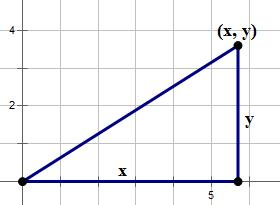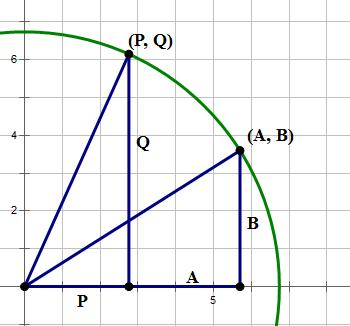# A Geometric and Visual Approach

Sometimes, the best choice from among two options is neither of them!

## Sample Problem

Q1. A, B, P, and Q are four positive numbers. Does AQ = BP?

Statement #1: \sqrt{A^2 + B^2} = \sqrt{P^2 + Q^2}

Statement #2: In the x-y plane, the line through (A, B) and (P, Q) goes through the origin.

You may want to wrestle with this a bit before reading on.

## Sample Problem Solution

Big geometry idea #1: every point in the x-y plane is the vertex of a unique little right triangle with legs parallel to the axes, with a second vertex on the x-axis and the third vertex at the origin. The length of the vertical leg is the y-coordinate, and the length of the horizontal leg is the x-coordinate.That one fact has several enormous implications. Notice, the distance from the point (x,y) to the origin is the hypotenuse, so we could find that distance with the Pythagorean Theorem.

distance = \sqrt{x^2 + y^2}

This should look familiar. That’s exactly the form we have for points (A, B) and (P, Q) in statement #1. The statement:

\sqrt{A^2 + B^2} = \sqrt{P^2 + Q^2}

is entirely equivalent to the statement that (A, B) and (P, Q) are equal distances from the origin. In other words, if we made a circle with center at the origin, we know both points would lie on the same circle.Well, how does this help us answer the question: Does AQ = BP? At least in the diagram as it is currently drawn, A and Q are the “big” legs and P and B are the “small” legs, and (big)*(big) \not = (small)*(small). For this example, clearly AQ \not = BP - a “no” answer to the prompt. But, if A = P and B = Q, the two points would be at the same place. Statement #1 would still be satisfied, and the answer to the prompt would then be “yes.” Statement #1 is consistent with both a “yes” answer and “no” answer to the prompt, so by itself it is insufficient.

Now, forget about Statement #1 and focus exclusively on Statement #2. We are told the line through (A, B) and (P, Q) goes through the origin, like this:

Big geometry idea #2: Two triangles of the same shape and same angles but different sizes are called similar. Similar triangles have proportional sides!! That is one of the deepest and most powerful ideas in all of geometry, and it has staggering implications for problem-solving on the QUANT.

Because the two right triangles are obvious similar, we can set up a proportion among the four sides of interest:

\dfrac{A}{B} = \dfrac{P}{Q}

Now, cross-multiply:

AQ = PB

Voila! It turns out: the prompt question is a proportionality question in disguise. (will you remember that trick on the QUANT?) Statement #2 allows us to answer the prompt with a definitive “Yes!”, so it is sufficient.